Home Practice
For learners and parents For teachers and schools
Textbooks
Full catalogue
Pricing SupportLog in

We think you are located in United States. Is this correct?

# Angles on a straight line

## 12.2 Angles on a straight line

Let us look at angles formed on one side of a straight line.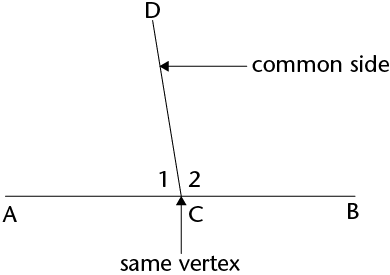In this diagram, line segment $$AB$$ meets line segment $$DC$$. The angle at the vertex, $$C$$, where they meet, is now split into two angles: $$\hat{C_1}$$ and $$\hat{C_2}$$.

$$\hat{C_1}$$ is the name for the angle at vertex $$C$$ labelled "1" (or $$A\hat{C}D$$).

### The sum of the angles formed on a straight line

The sum of the angles that are formed on a straight line is always $$180^{\circ}$$.

We can shorten this property as: $$\angle$$s on a straight line.

• Two angles that add up to $$180^{\circ}$$ are called supplementary angles.
• Angles that share a vertex and a common side are said to be adjacent angles. $$\hat{C_1}$$ and $$\hat{C_2}$$ are supplementary angles.
• Hence, $$\hat{C_1}$$ and $$\hat{C_2}$$ are called adjacent supplementary angles.
supplementary angles
two angles that add up to $$180^{\circ}$$
angles that share a vertex and a common side

You can have more than one line meeting at the same point on a straight line. Here are a few examples of angles on a straight line.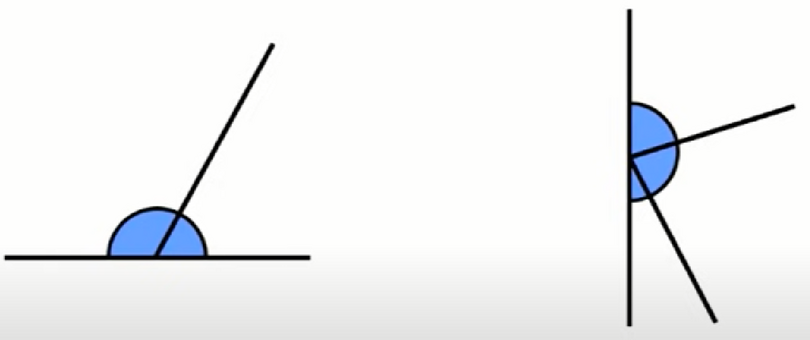### The sum of the angles on perpendicular lines

When two lines are perpendicular, the adjacent supplementary angles are both equal to $$90^{\circ}$$.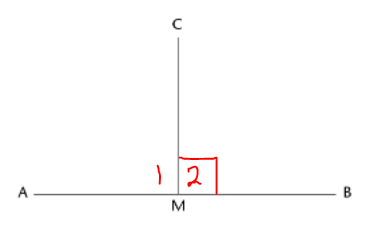In the diagram, $$\hat{M_1}=\hat{M_2} =90^{\circ}$$.

A right angle is shown by forming a square at one of the right angles, like this: ⦜.

## Worked example 12.1: Calculating unknown angles on a straight line

Calculate the size of $$x$$.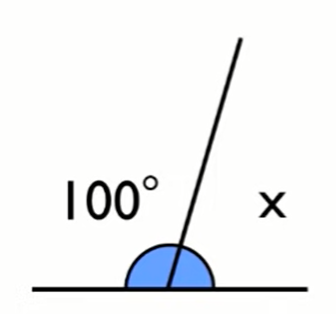In the diagram, we have two angles that are on the same side of the straight line. The first angle is $$100^{\circ}$$ and the second angle is unknown ($$x$$). We need to calculate the size of $$x$$.

### We know that the two angles have a sum of $$180^{\circ}$$, so we can say that:

$$100^{\circ}+x=180^{\circ}$$ ($$\angle$$s on a straight line)

### Now we can solve this equation.

\begin{align} x&=180^{\circ}-100^{\circ} \\ x&=80^{\circ} \end{align}

## Worked example 12.2: Calculating unknown angles on a straight line

Calculate the size of $$x$$.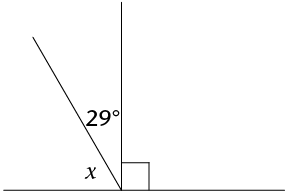### Notice that there are three angles on the same side of the straight line. We have $$x$$, an angle of $$29^{\circ}$$ and an angle of $$90^{\circ}$$. (Remember that the ⦜ symbol on the diagram indicates a $$90^{\circ}$$ angle.) These three angles have a sum of $$180^{\circ}$$, so we can say that:

$$x+29^{\circ}+90^{\circ}=180^{\circ}$$ ($$\angle$$s on a straight line)

### Now we can solve this equation.

\begin{align} x+29^{\circ}+90^{\circ}&=180^{\circ} \\ x+119^{\circ}&=180^{\circ} \\ x&=180^{\circ}-119^{\circ} \\ x&=61^{\circ} \end{align}

### There is a simpler way to solve for $$x$$. It is given that we have a perpendicular line. Adjacent angles on a perpendicular line are both equal to $$90^{\circ}$$. So, we have a different equation to solve.

\begin{align} x+29^{\circ}&=90^{\circ} \\ x&=90^{\circ}-29^{\circ} \\ x&=61^{\circ} \end{align}

## Worked example 12.3: Calculating unknown angles on a straight line

Calculate the size of $$y$$.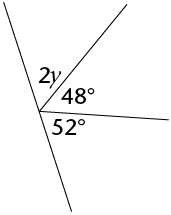### Notice that we have three angles on the same side of the straight line. We have $$2y$$, an angle of $$48^{\circ}$$ and an angle of $$52^{\circ}$$. These three angles have a sum of $$180^{\circ}$$, so we can say that:

$$2y+48^{\circ}+52^{\circ}=180^{\circ}$$ ($$\angle$$s on a straight line)

### Now we can solve this equation.

\begin{align} 2y+48^{\circ}+52^{\circ}&=180^{\circ} \\ 2y+100^{\circ}&=180^{\circ} \\ 2y&=180^{\circ}-100^{\circ} \\ 2y&=80^{\circ} \\ y&=40^{\circ} \end{align}
Exercise 12.1

Calculate the size of $$a$$.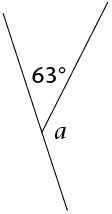\begin{align} a+63^{\circ}&=180^{\circ} &(\angle\text{s on a straight line}) \\ x&=180^{\circ}-63^{\circ} \\ x&=117^{\circ} \end{align}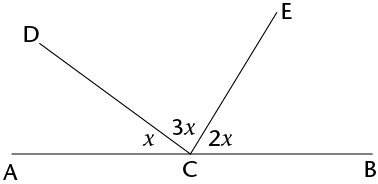Calculate the size of:

1. $$x$$
2. $$\hat{ECB}$$
1. \begin{align} x+3x+2x&=180^{\circ} &(\angle\text{s on a straight line}) \\ 6x&=180^{\circ} \\ x&=30^{\circ} \end{align}
2. \begin{align} \hat{ECB}&=2x \\ &=2(30^{\circ})\\ &=60^{\circ} \end{align}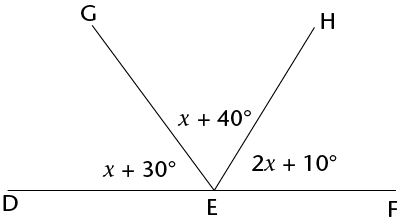Calculate the size of:

1. $$x$$
2. $$\hat{GEH}$$
1. \begin{align} (x+30^{\circ})+(x+40^{\circ}) +(2x+10^{\circ}) &=180^{\circ} &(\angle\text{s on a straight line}) \\ 4x+80^{\circ}&=180^{\circ} \\ 4x&=100^{\circ} \\ x&=25^{\circ} \end{align}
2. \begin{align} \hat{GEH}&=x+40^{\circ} \\ &=25^{\circ}+40^{\circ} \\ &=65^{\circ} \end{align}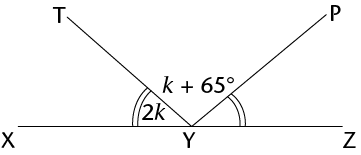Hint: Remember that the matching curved lines “))” indicate that the angles are equal.

Calculate the size of:

1. $$k$$
2. $$\hat{TYP}$$
1. \begin{align} (2k)+(k+65^{\circ}) +(2k) &=180^{\circ} &&(\angle\text{s on a straight line}) \\ 5k+65^{\circ}&=180^{\circ} \\ 5k &=115^{\circ} \\ &=23^{\circ} \end{align}
2. \begin{align} \hat{TYP}&=k+65^{\circ} \\ &=23^{\circ}+65^{\circ} \\ &=88^{\circ} \end{align}